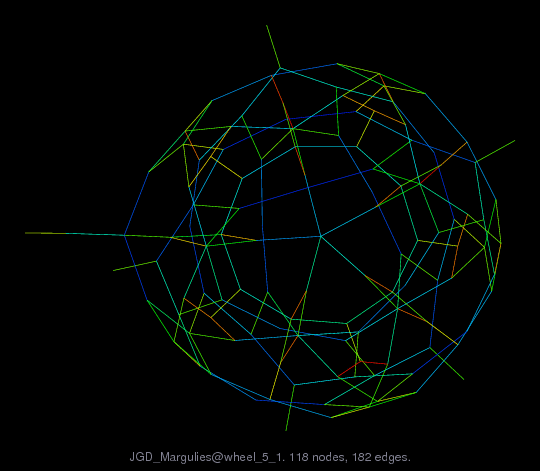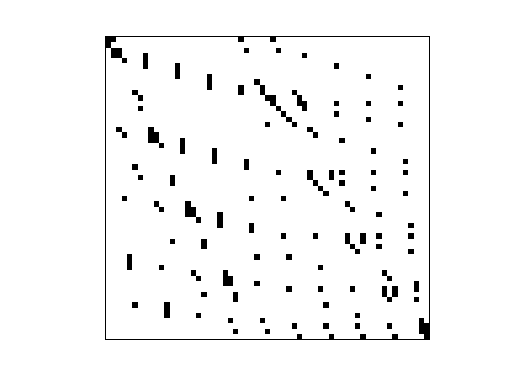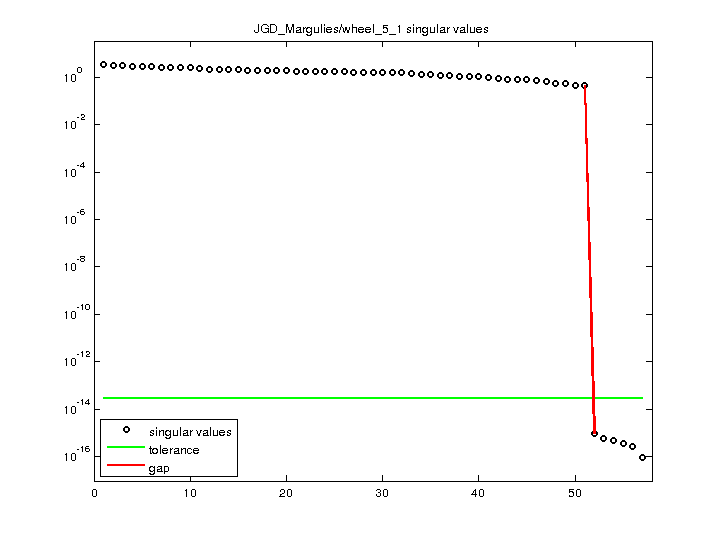Matrix: JGD_Margulies/wheel_5_1

Description: Combinatorial optimization as polynomial eqns, Susan Margulies, UC Davis(bipartite graph drawing)• Matrix group: JGD_Margulies
• download as a MATLAB mat-file, file size: 2 KB. Use UFget(2168) or UFget('JGD_Margulies/wheel_5_1') in MATLAB.

 Matrix properties number of rows 57 number of columns 61 nonzeros 182 structural full rank? yes structural rank 57 # of blocks from dmperm 12 # strongly connected comp. 1 explicit zero entries 0 nonzero pattern symmetry 0% numeric value symmetry 0% type binary structure rectangular Cholesky candidate? no positive definite? no

 author S. Margulies editor J.-G. Dumas date 2008 kind combinatorial problem 2D/3D problem? no

Notes:

```Combinatorial optimization as polynomial eqns, Susan Margulies, UC Davis
From Jean-Guillaume Dumas' Sparse Integer Matrix Collection,
http://ljk.imag.fr/membres/Jean-Guillaume.Dumas/simc.html

http://arxiv.org/abs/0706.0578

Expressing Combinatorial Optimization Problems by Systems of Polynomial
Equations and the Nullstellensatz

Authors: J.A. De Loera, J. Lee, Susan Margulies, S. Onn

(Submitted on 5 Jun 2007)

Abstract: Systems of polynomial equations over the complex or real
numbers can be used to model combinatorial problems. In this way, a
combinatorial problem is feasible (e.g. a graph is 3-colorable,
hamiltonian, etc.) if and only if a related system of polynomial
equations has a solution. In the first part of this paper, we construct
new polynomial encodings for the problems of finding in a graph its
longest cycle, the largest planar subgraph, the edge-chromatic number,
or the largest k-colorable subgraph.  For an infeasible polynomial
system, the (complex) Hilbert Nullstellensatz gives a certificate that
the associated combinatorial problem is infeasible. Thus, unless P =
NP, there must exist an infinite sequence of infeasible instances of
each hard combinatorial problem for which the minimum degree of a
Hilbert Nullstellensatz certificate of the associated polynomial system
grows.  We show that the minimum-degree of a Nullstellensatz
certificate for the non-existence of a stable set of size greater than
the stability number of the graph is the stability number of the graph.
Moreover, such a certificate contains at least one term per stable set
of G. In contrast, for non-3- colorability, we found only graphs with
Nullstellensatz certificates of degree four.

Filename in JGD collection: Margulies/wheel_5_1.sms
```

 Ordering statistics: result nnz(V) for QR, upper bound nnz(L) for LU, with COLAMD 290 nnz(R) for QR, upper bound nnz(U) for LU, with COLAMD 445

 SVD-based statistics: norm(A) 3.46779 min(svd(A)) 9.15158e-17 cond(A) 3.78928e+16 rank(A) 51 sprank(A)-rank(A) 6 null space dimension 6 full numerical rank? no singular value gap 5.30858e+14

 singular values (MAT file): click here SVD method used: s = svd (full (A)) ; status: ok